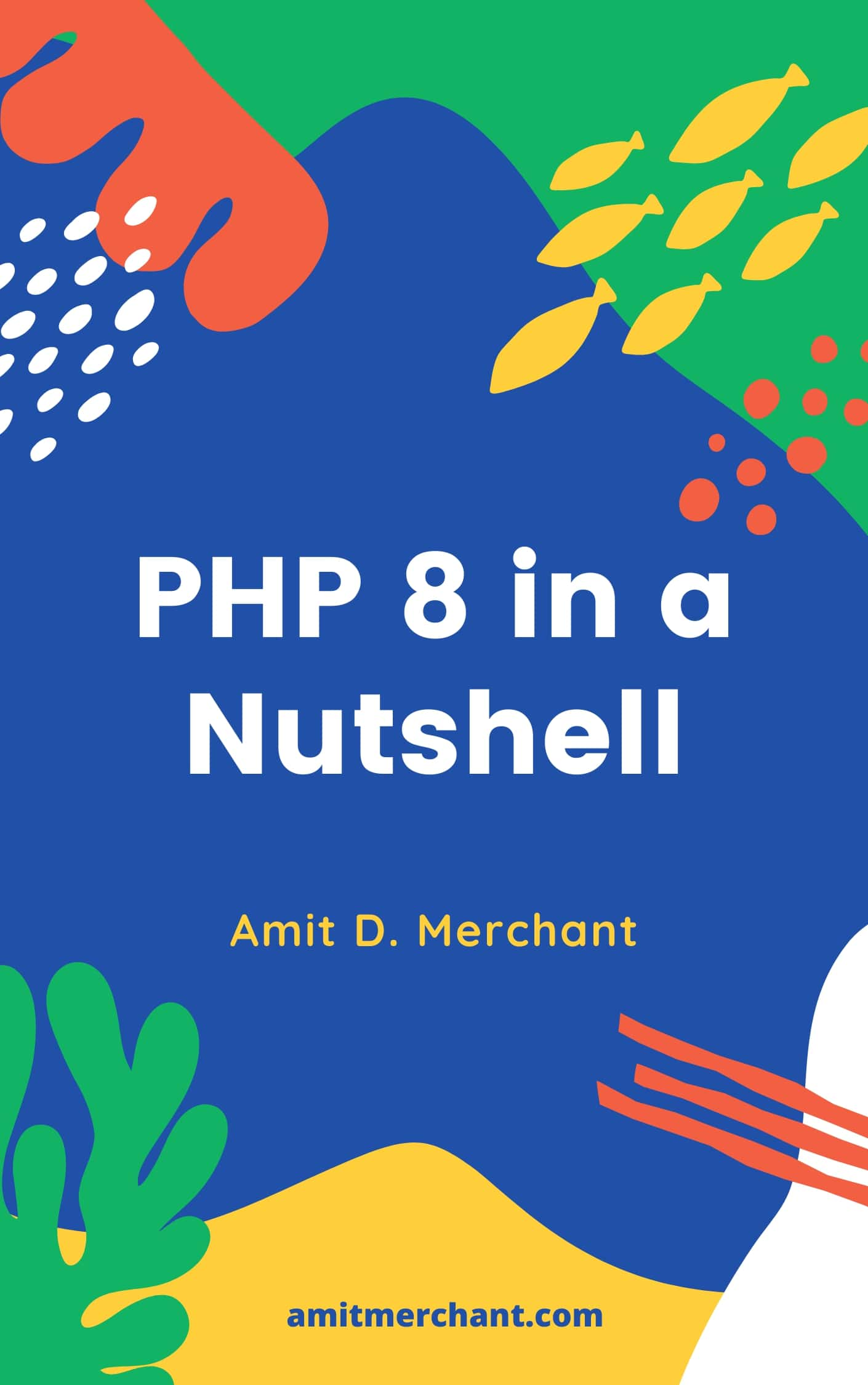# Amit MerchantVerified (\$10/year for the domain)

A blog on PHP, JavaScript, and more

# How to group where constraints in Laravel Eloquent

When writing MySQL queries, if there are more than two where conditions, you’d want to group certain constraints/conditions to avoid unexpected behavior. For instance, check the following query.

``````select * from books
where author_name = 'JK Rowling'
and rating > 4
or genre = 'fiction';
``````

Here, if we don’t specifically mention how the `and` and `or` conditions should get evaluated, we might get unexpected results. So, we must explicitly determine their order and for that and in MySQL, we can use parenthesis to achieve this.

If we want to determine if `rating > 4 or genre = 'fiction'` in the previous query should get evaluated first, we can do it using parenthesis like so.

``````select * from books
where author_name = 'JK Rowling'
and (rating > 4 or genre = 'fiction');
``````

We can achieve a similar thing in Eloquent as well. For this, we can pass in a `Closure` into the `where` method of Eloquent where we can further set the constraints which want to be grouped. So, if we want to write the previous query using Eloquent, we can do it like so.

``````\$books = Book::where('author_name', '=', 'JK Rowling')
->where(function (\$query) {
\$query->where('rating', '>', 4)
->orWhere('genre', '=', 'fiction');
})
->get();
``````

As you can see here, we passed in a `Closure` to the `where` condition which will receive a query builder instance `\$query` on which we can add more constraints which will be contained within the parenthesis group.

👋 Hi there! I'm Amit. I write articles about all things web development. If you like what I do and want me to continue doing the same, I'd like you consider leaving a tip. I'd highly appreciate that. Cheers!(Includes PHP 8.1 and 8.2)

My Tiny YouTube Channel ➔